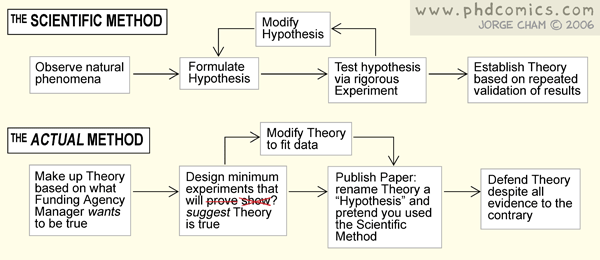# CSCE 145: Practice Test 1

1. Draw a UML class diagram for the following class. Make sure to also include any direct superclass (but, no details are needed for the superclass).
```import becker.robots.City;
import becker.robots.Direction;
import becker.robots.Robot;

public class MyRobot extends Robot {

private Direction lookingDirection;

public MyRobot(City arg0, int arg1, int arg2, Direction arg3) {
super(arg0, arg1, arg2, arg3);
// TODO Auto-generated constructor stub
}

public static void main(String[] args) {
// TODO Auto-generated method stub

}

private int money;

public void findTreasure(int x, int y){

}

public ThingBag purse;

private int numPhonesInPurse(){

}

}
```
2. In the preceding program
1. Which one is the constructor?
2. Which one is the parent class?
3. What are the arguments the constructor takes?
4. How many attributes does the MyRobot class have?
5. Which services does the MyRobot class make available to others?
6. Write the line of code you need to create an instance of MyRobot.
3. Write a function called isOnItems which takes one integer argument x and returns true if there are at least x items on the robot's current intersection. The function should have the properly formed javadoc comment.
4. Write a class which over-writes Robot's turnLeft() function and makes him move forward instead (obviously, this is a bad idea).
5. Where does steve end up after running the following program?
```import becker.robots.*;

public class Wanderer {

public static void main(String[] args) {
City columbia = new City();
RobotSE steve  = new RobotSE(columbia, 0, 0, Direction.SOUTH);
int marbles = 5;
while (marbles > 3){
steve.move();
marbles = marbles - 1;
};
steve.turnLeft();
steve.move(marbles);
}

}
```
6. Find and explain all the syntax errors in this program:
```import becker.robots.*;

City columbia = new City();
Robot robby = new Robot(columbia, 0, 0, SOUTH);

while (i < 10){
//do something
}

super();
}

private static void main(String[] args) {
steve = new Robot(charlotte,0,0,Direction.NORTH;)
turnLeft();
}

}
```
7. Implement a robot which, given a height and a base numbers, places flowers (Things) such that they form a triangle of base width = base and height = height.Jose M Vidal